# Bayesian Inference on a Pendulum using Turing.jl

### Set up simple pendulum problem

using DiffEqBayes, OrdinaryDiffEq, RecursiveArrayTools, Distributions, Plots, StatsPlots


Let's define our simple pendulum problem. Here our pendulum has a drag term ω and a length L.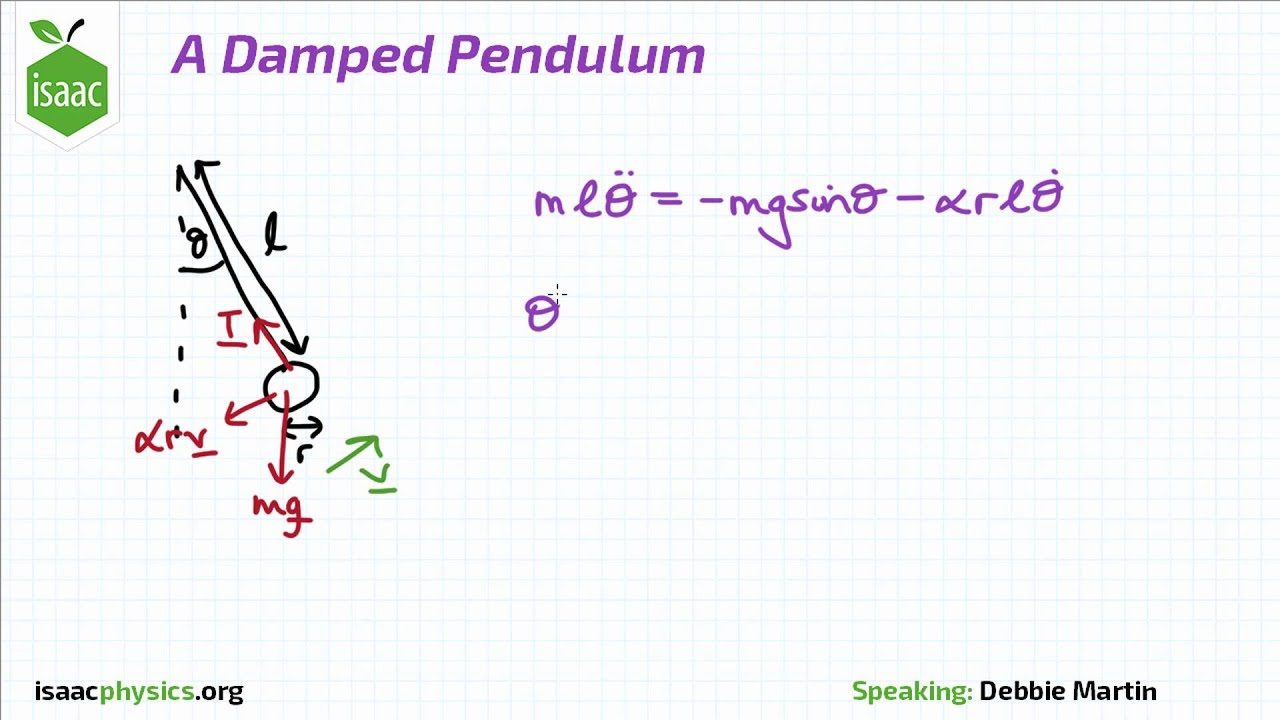We get first order equations by defining the first term as the velocity and the second term as the position, getting:

function pendulum(du,u,p,t)
ω,L = p
x,y = u
du = y
du = - ω*y -(9.8/L)*sin(x)
end

u0 = [1.0,0.1]
tspan = (0.0,10.0)
prob1 = ODEProblem(pendulum,u0,tspan,[1.0,2.5])

ODEProblem with uType Array{Float64,1} and tType Float64. In-place: true
timespan: (0.0, 10.0)
u0: [1.0, 0.1]


### Solve the model and plot

To understand the model and generate data, let's solve and visualize the solution with the known parameters:

sol = solve(prob1,Tsit5())
plot(sol)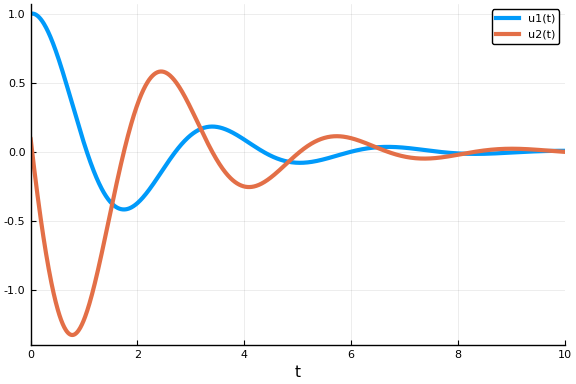It's the pendulum, so you know what it looks like. It's periodic, but since we have not made a small angle assumption it's not exactly sin or cos. Because the true dampening parameter ω is 1, the solution does not decay over time, nor does it increase. The length L determines the period.

### Create some dummy data to use for estimation

We now generate some dummy data to use for estimation

t = collect(range(1,stop=10,length=10))
randomized = VectorOfArray([(sol(t[i]) + .01randn(2)) for i in 1:length(t)])
data = convert(Array,randomized)

2×10 Array{Float64,2}:
0.0615493  -0.368933  0.128309   0.0971307  …  -0.0205744  -0.00052941
-1.21598     0.365816  0.31529   -0.252625       0.0151109  -0.00473605


Let's see what our data looks like on top of the real solution

scatter!(data')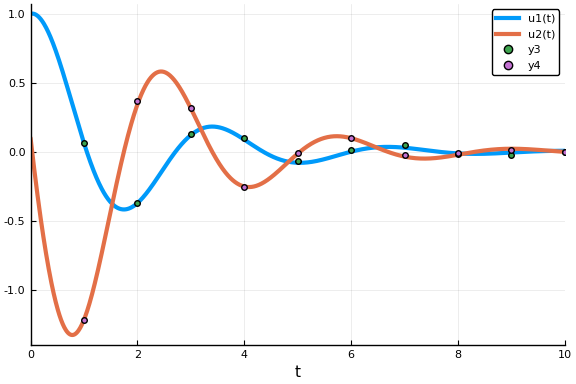This data captures the non-dampening effect and the true period, making it perfect to attempting a Bayesian inference.

### Perform Bayesian Estimation

Now let's fit the pendulum to the data. Since we know our model is correct, this should give us back the parameters that we used to generate the data! Define priors on our parameters. In this case, let's assume we don't have much information, but have a prior belief that ω is between 0.1 and 3.0, while the length of the pendulum L is probably around 3.0:

priors = [Uniform(0.1,3.0), Normal(3.0,1.0)]

2-element Array{Distributions.Distribution{Distributions.Univariate,Distrib
utions.Continuous},1}:
Distributions.Uniform{Float64}(a=0.1, b=3.0)
Distributions.Normal{Float64}(μ=3.0, σ=1.0)


Finally let's run the estimation routine from DiffEqBayes.jl using the Turing.jl backend

bayesian_result = turing_inference(prob1,Tsit5(),t,data,priors;num_samples=10_000,
syms = [:omega,:L])

Object of type Chains, with data of type 10000×4×1 Array{Union{Missing, Flo
at64},3}

Log evidence      = -3.4208122518018893
Iterations        = 1:10000
Thinning interval = 1
Chains            = 1
Samples per chain = 10000
internals         = lp
parameters        = _theta, σ, _theta

2-element Array{MCMCChains.ChainDataFrame,1}

Summary Statistics
. Omitted printing of 1 columns
│ Row │ parameters │ mean    │ std      │ naive_se   │ mcse       │ ess
│
│     │ Symbol     │ Float64 │ Float64  │ Float64    │ Float64    │ Any
│
├─────┼────────────┼─────────┼──────────┼────────────┼────────────┼────────
─┤
│ 1   │ _theta  │ 1.54937 │ 0.837743 │ 0.00837743 │ 0.00791079 │ 10000.0
│
│ 2   │ _theta  │ 3.02832 │ 1.00472  │ 0.0100472  │ 0.00923376 │ 10000.0
│
│ 3   │ σ          │ 2.89973 │ 5.31736  │ 0.0531736  │ 0.053681   │ 10000.0
│

Quantiles

│ Row │ parameters │ 2.5%     │ 25.0%    │ 50.0%   │ 75.0%   │ 97.5%   │
│     │ Symbol     │ Float64  │ Float64  │ Float64 │ Float64 │ Float64 │
├─────┼────────────┼──────────┼──────────┼─────────┼─────────┼─────────┤
│ 1   │ _theta  │ 0.171025 │ 0.826122 │ 1.551   │ 2.26978 │ 2.92857 │
│ 2   │ _theta  │ 1.07599  │ 2.35301  │ 3.00239 │ 3.7014  │ 5.0227  │
│ 3   │ σ          │ 0.538211 │ 1.10267  │ 1.75954 │ 3.06687 │ 11.9888 │


Notice that while our guesses had the wrong means, the learned parameters converged to the correct means, meaning that it learned good posterior distributions for the parameters. To look at these posterior distributions on the parameters, we can examine the chains:

plot(bayesian_result)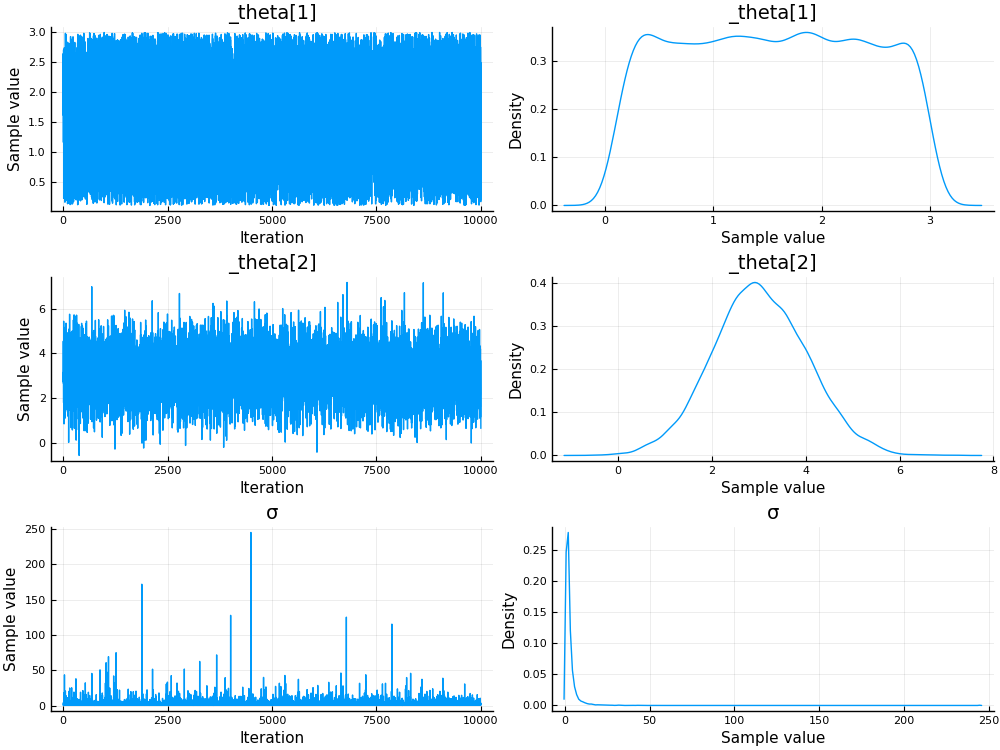As a diagnostic, we will also check the parameter chains. The chain is the MCMC sampling process. The chain should explore parameter space and converge reasonably well, and we should be taking a lot of samples after it converges (it is these samples that form the posterior distribution!)

plot(bayesian_result, colordim = :parameter)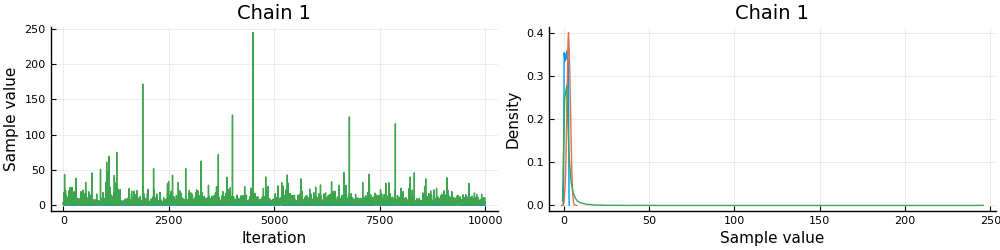Notice that after awhile these chains converge to a "fuzzy line", meaning it found the area with the most likelihood and then starts to sample around there, which builds a posterior distribution around the true mean.